Next: Laser Up: Physics and Numerical Methods Previous: Luminosity Integration

## Beam Field

One of the basic assumptions of CAIN is that the most particles in the beams have high energy and are almost either right- or left-going. This assumption leads to the following facts:
• A field due to a particle is almost concentrated in a transverse plane with the same s-coordinate of the particle because of the Lorentz contraction.
• If the electric field is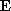, the magnetic field is given by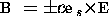, where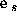is the unit vector along the s-axis, c the velocity of light, and the upper (lower) sign is for the field created by the right(left)-goin beam.
In contrast to ABEL, CAIN does not assume that all the particles have the above property. Some particles may have low energies and large angles with respect to the s-axis. CAIN will work, unless the sum of their weight becomes a significant fraction of the beam. The equation of motion under the Lorentz force is integrated with possible low energies and large angles taken into account.

The calculation of the beam electric field is done in the following way. First, cut the right(left)-going particles into longitudinal slices (the width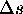is defined by the parameter Smesh).    Within each slice the following Poisson equation  is solved.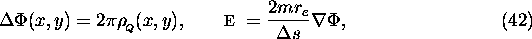where m is the electron mass in units of eV/c,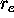the classical electron radius in meters,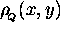is the charge (divided by the elementary charge) per unit transverse area, thenis given in units of V/m.

For each slice and for each of right- and left going beams, a region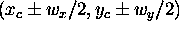is selected, where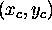is the center-of-mass and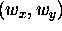is the width determined by the input parameters. The field created by the particles outside this region is ignored. Let us name this region [O].

In the region [O], the Poisson equation  is solved using the FFT. Eq.(42) can formally be solved as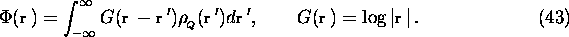Divide this region by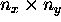grid. Within each cell (i,j) (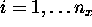,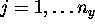), the the densityis approximated by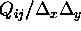, where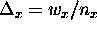,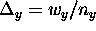, and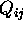is the total charge in the cell: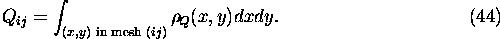where (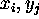) is the cell center coordinate. Then, eq.(43) becomes a sum over the cells.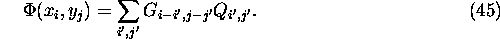The kernel matrix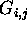has to be calculated by taking average over the source cell: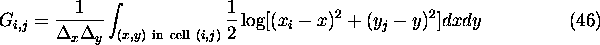This averaging is important when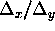is far from unity. The convolution in eq.(45) can be done efficiently by using FFT.

However, if we apply FFT for the finite regioninstead of the infinite region in eq.(43), we would be assuming a periodic charge distribution, i.e., the charge distribution inis infinitely repeated. To avoid this problem, we use the following trick. First double the region to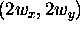by padding zero in the extended region and carry out FFT. This still means a periodic charge distribution as depicted in Fig.3. However, if we use the kernel matrix with zero padded   in the extended region (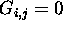if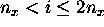or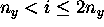), the field due to the ghost charges will never reach the real charge region because their horizontal(vertical) distance is larger than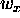(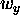). Thus, the potential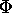in the regionis calculated correctly although incorrect in the extended region.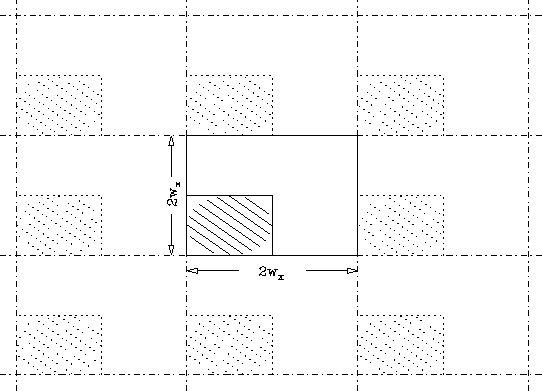Figure 3: Doubled region for FFT. The solid frame indicates the doubled region for FFT and its left-bottom quadrant is the charge region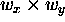. The region hatched by solid lines is the real charge region and that by dotted lines the ghost charge due to the periodicity of Fourier transformation.

The obtained values of the potential are those at cell centers. They are interpolated by 2-dimensional cubic spline and differentiated to get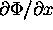and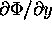.

When a charged particle gets out of the mesh region, the field created by it is ignored in CAIN. However, the force by the other beam is taken into account even if the particle is outside the mesh region of the other beam. To this end, CAIN  adopts three methods, namely, [A] direct Coulomb force by the charge distribution in the mesh, [B] harmonic expansion in polar coordinate, and [C] harmonic expansion in elliptic coordinate.

Letbe the total width of the mesh region. If it is close to a square, or more precisely, if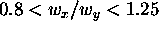, the whole region is divided into three regions [O],[A],[B], as depicted in Fig.4a. If the mesh region is far from square, the whole region is divided into four, [O],[A],[B],[C], as in Fig.4b. In the region [O] the mesh is used for calculating the field. In other regions, the methods mentioned above are used.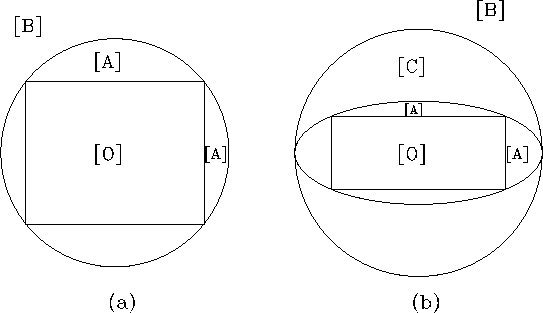Figure 4: Regions for calculating the beam field

Since the sum is time consuming, this is used only in region [A], where two other methods fail to converge. The method is trivial and given by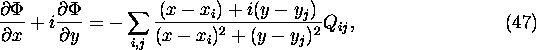However, this formula is not accurate when the bin size ratiois far from unity. It is needed to take average over a bin when the bin is close to the field point (x,y). CAIN makes a table for the Coulomb force by a bin (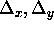) for faster computation.

In the region [B] the following formula is used.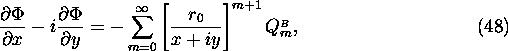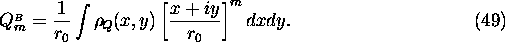Here,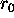is arbitrary (introduced for avoiding overflow/underflow). The formula is valid for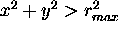, where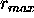=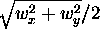is the maximum radius of the mesh region.

When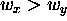(otherwise, exchange x and y), the elliptic coordinate (u,v) defined by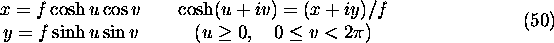is used. Here, f is chosen as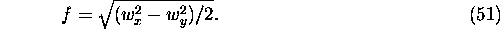The maximum of the radial-like coordinate u in the mesh region is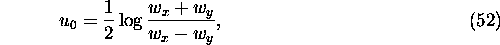which is taken at the four corners.

Then, the expansion ofis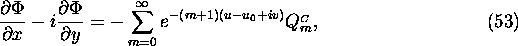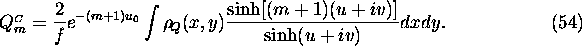Actually, there is a finite relation between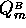and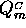: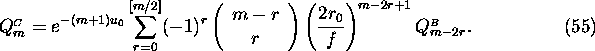The formula converges if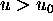, which corresponds to the region [C] (and [B]) in Fig.4b. The truncation of the series is defined by the operand NMOM of the command BBFIELD (common to the two types of expansions for simplicity).Next: Laser Up: Physics and Numerical Methods Previous: Luminosity Integration

Toshiaki Tauchi
Thu Dec 3 17:27:26 JST 1998